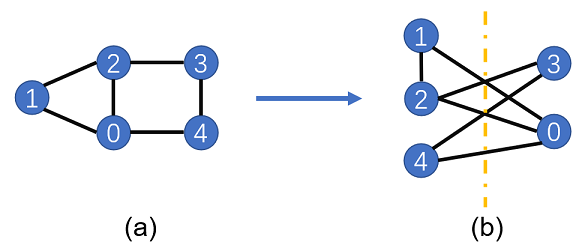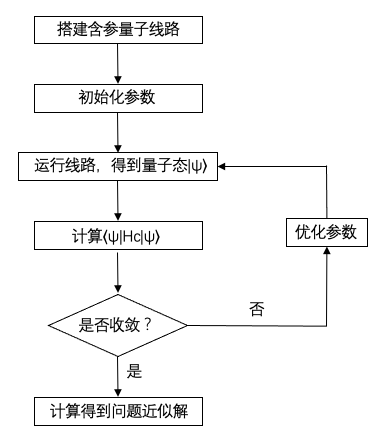# 量子近似优化算法

## 环境准备

• networkx

networkx是创建、操作和研究复杂网络的结构、动态和功能库。可通过如下代码来进行安装。

pip3 install networkx


## Max-Cut问题描述

Max-Cut问题是图论中的一个NP-complete问题，它需要将一个图中的顶点分成两部分，并使得两部分被切割的边最多。如下图（a），一个图由五个顶点构成，相互连接的边为(0, 1), (0, 2), (1, 2), (2, 3), (3, 4), (0, 4)。为了使得被切割的边最多，我们尝试通过（b）图的分割，将1、2、4分为一组，0、3分成另一组，因此可得到被切割的边有5条。后面我们将用穷举法验证这个解是否正确。当图中顶点较少时，我们可以在较短时间内通过穷举法找到最大的切割边数，但当图中顶点增多时，我们很难找到有效的经典算法来解决Max-Cut问题，因为这类NP-complete问题很有可能不存在多项式时间算法。但尽管精确解不容易得到，我们却可以想办法在多项式时间内找到问题的一个近似解，这就是近似优化算法。下面，我们介绍怎么将Max-Cut问题转化为一个哈密顿量的基态能量求解问题。## Max-Cut问题量子化

$\langle 01|H|01\rangle=-1,\quad \langle 10|H|10\rangle=-1$

$\langle 00|H|00\rangle=0,\quad \langle 11|H|11\rangle=0$

$Z_1Z_0|00\rangle=|00\rangle,\quad Z_1Z_0|11\rangle=|11\rangle, \quad Z_1Z_0|01\rangle=-|01\rangle, \quad Z_1Z_0|10\rangle=-|10\rangle$

$\left<01\right|H\left|01\right>=\frac{1}{2}\left<01\right|Z_1Z_0\left|01\right>-\frac{1}{2}=-1$
$\left<10\right|H\left|10\right>=\frac{1}{2}\left<10\right|Z_1Z_0\left|10\right>-\frac{1}{2}=-1$

$\left<00\right|H\left|00\right>=\frac{1}{2}\left<00\right|Z_1Z_0\left|00\right>-\frac{1}{2}=0$
$\left<11\right|H\left|11\right>=\frac{1}{2}\left<11\right|Z_1Z_0\left|11\right>-\frac{1}{2}=0$

$H=\sum_{(i,j)\in C}(Z_iZ_j-1)/2$

## 导入相关依赖

:

from mindquantum.core.circuit import Circuit, UN
from mindquantum.core.gates import H, Rzz, RX
from mindquantum.core.operators import Hamiltonian, QubitOperator
from mindquantum.framework import MQAnsatzOnlyLayer
from mindquantum.simulator import Simulator
import networkx as nx
import mindspore.nn as nn


## 搭建所需求解的图

:

g = nx.Graph()
nx.draw(g, with_labels=True, font_weight='bold'):

for i in g.nodes:
print('one size:', [i], 'cut=', nx.cut_size(g, [i]))            # 一组1个节点、另一组4个节点的所有情况
for j in range(i):
print('one size:', [i, j], 'cut=', nx.cut_size(g, [i, j]))  # 一组2个节点、另一组3个节点的所有情况

one size:  cut= 3
one size:  cut= 2
one size: [1, 0] cut= 3
one size:  cut= 3
one size: [2, 0] cut= 4
one size: [2, 1] cut= 3
one size:  cut= 2
one size: [3, 0] cut= 5
one size: [3, 1] cut= 4
one size: [3, 2] cut= 3
one size:  cut= 2
one size: [4, 0] cut= 3
one size: [4, 1] cut= 4
one size: [4, 2] cut= 5
one size: [4, 3] cut= 2


## QAOA算法整体流程

1. 搭建QAOA量子线路，其中ansatz线路包含可以训练的参数

2. 初始化线路中的参数

3. 运行该量子线路，得到量子态$$|\psi\rangle$$

4. 计算目标哈密顿量$$H_C$$的期望值$$\langle\psi|H_C|\psi\rangle$$

6. 重复3-5步，直到第4步结果基本不再变化

7. 根据第4步的结果，算出目标问题的近似解## 搭建QAOA量子线路

$H_C=\sum_{(i,j)\in C}(Z_iZ_j-1)/2$

$H_B=\sum_i X_i$

### ansatz线路

$H_B=\sum_i X_i$

$H(t)=(1-\frac{t}{T})H_B+(\frac{t}{T})H_C$

$|\psi (T)\rangle=\mathcal{T}e^{-i\int^{T}_{0} H(t)dt}|\psi(0)\rangle$

$\mathcal{T}e^{-i\int^T_0 H(t)dt}=\lim_{N\rightarrow \infty}\prod^N_{l=1}e^{-iH(t_l)\Delta t},\quad \Delta t=\frac{T}{N},\quad t_l=l\Delta t$

$\mathcal{T}e^{-i\int^T_0 H(t)dt}\approx \lim_{N\rightarrow \infty}\prod^N_{l=1}e^{-iH_B(1-t_l/T)\Delta t}e^{-iH_C t_l\Delta t/T}$

$$\beta_l=(1-t_l/T)\Delta t$$$$\gamma_l=t_l\Delta t/T$$，并取$$N$$为一个有限大的整数，即得到QAOA的ansatz

$|\psi(\gamma,\beta)\rangle=\prod^p_{l=1}e^{-i\beta_l H_B}e^{-i\gamma_l H_C}|\psi_{in}\rangle$

:

def build_hc(g, para):
hc = Circuit()                  # 创建量子线路
for i in g.edges:
hc += Rzz(para).on(i)        # 对图中的每条边作用Rzz门
hc.barrier()                    # 添加Barrier以方便展示线路
return hc


:

# pylint: disable=W0104
circuit = build_hc(g, 'gamma')
circuit.svg()

::

def build_hb(g, para):
hb = Circuit()                  # 创建量子线路
for i in g.nodes:
hb += RX(para).on(i)        # 对每个节点作用RX门
hb.barrier()                    # 添加Barrier以方便展示线路
return hb


:

# pylint: disable=W0104
circuit = build_hb(g, 'beta')
circuit.svg()

::

# pylint: disable=W0104
circuit = build_hc(g, 'gamma') + build_hb(g, 'beta')
circuit.svg()

::

def build_ansatz(g, p):                    # g是max-cut问题的图，p是ansatz线路的层数
circ = Circuit()                       # 创建量子线路
for i in range(p):
circ += build_hc(g, f'g{i}')       # 添加Uc对应的线路，参数记为g0、g1、g2...
circ += build_hb(g, f'b{i}')       # 添加Ub对应的线路，参数记为b0、b1、b2...
return circ


:

def build_ham(g):
ham = QubitOperator()
for i in g.edges:
ham += QubitOperator(f'Z{i} Z{i}')  # 生成哈密顿量Hc
return ham


### 生成完整的量子线路和图所对应的哈密顿量

:

# pylint: disable=W0104
p = 4
ham = Hamiltonian(build_ham(g))              # 生成哈密顿量
init_state_circ = UN(H, g.nodes)             # 生成均匀叠加态，即对所有量子比特作用H门
ansatz = build_ansatz(g, p)                  # 生成ansatz线路
circ = init_state_circ + ansatz              # 将初始化线路与ansatz线路组合成一个线路
circ.svg()

:### 搭建待训练量子神经网络

:

import mindspore as ms
ms.set_context(mode=ms.PYNATIVE_MODE, device_target="CPU")

sim = Simulator('mqvector', circ.n_qubits)                     # 创建模拟器，backend使用‘mqvector’，能模拟5个比特（'circ'线路中包含的比特数）
train_net = nn.TrainOneStepCell(net, opti)                     # 对神经网络进行一步训练


## 训练并展示结果

:

for i in range(200):
cut = (len(g.edges) - train_net()) / 2      # 将神经网络训练一步并计算得到的结果（切割边数）。注意：每当'train_net()'运行一次，神经网络就训练了一步
if i%10 == 0:
print("train step:", i, ", cut:", cut)  # 每训练10步，打印当前训练步数和当前得到的切割边数

train step: 0 , cut: [2.9993382]
train step: 10 , cut: [4.4533334]
train step: 20 , cut: [4.699728]
train step: 30 , cut: [4.783018]
train step: 40 , cut: [4.854207]
train step: 50 , cut: [4.8817496]
train step: 60 , cut: [4.9091177]
train step: 70 , cut: [4.9321637]
train step: 80 , cut: [4.93795]
train step: 90 , cut: [4.937838]
train step: 100 , cut: [4.939126]
train step: 110 , cut: [4.9390845]
train step: 120 , cut: [4.9392385]
train step: 130 , cut: [4.9392385]
train step: 140 , cut: [4.939252]
train step: 150 , cut: [4.9392557]
train step: 160 , cut: [4.9392567]
train step: 170 , cut: [4.939257]
train step: 180 , cut: [4.939257]
train step: 190 , cut: [4.939257]


### 最优参数

:

pr = dict(zip(ansatz.params_name, net.weight.asnumpy())) # 获取线路参数
print(pr)

{'g0': -0.44880474, 'b0': 1.1390473, 'g1': -0.90614676, 'b1': 0.94465256, 'g2': -1.0674977, 'b2': 0.67762387, 'g3': -1.1678733, 'b3': 0.38237205}


### 概率图

:

# pylint: disable=W0104
circ.measure_all()                               # 为线路中所有比特添加测量门
sim.sampling(circ, pr=pr, shots=1000).svg()      # 运行线路1000次并打印结果

:• 01001：编号为1、2、4的顶点在左边，编号为0、3的顶点在右边。

• 10110：编号为0、3的顶点在左边，编号为1、2、4的顶点在右边。

• 01011：编号为2、4的顶点在左边，编号为0、1、3的顶点在右边。

• 10100：编号为0、1、3的顶点在左边，编号为2、4的顶点在右边。

## 总结

:

from mindquantum.utils.show_info import InfoTable

InfoTable('mindquantum', 'scipy', 'numpy')

:

Software Version
mindquantum0.9.0
scipy1.10.1
numpy1.23.5
System Info
Python3.8.17
OSWindows AMD64
Memory8.39 GB